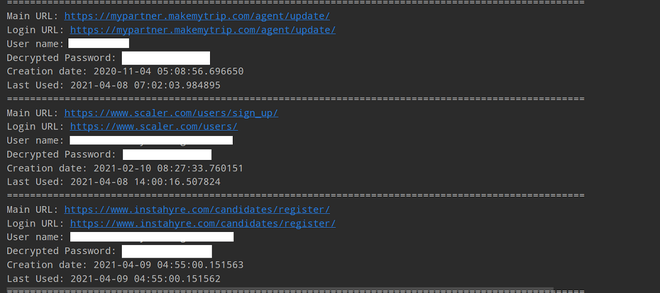Open in App
Not now

# How to Extract Chrome Passwords in Python?

• Last Updated : 21 Apr, 2021

Note: This article is for users who use Chrome on Windows. If you are a Mac or Linux user, you may need to make some changes to the given path, while the rest of the Python program will remain the same.

### Installation:

Now, Let’s install some important libraries which we need to write a python program through which we can extract Chrome Passwords.

```pip install pycryptodome
pip install pypiwin32```

Before we extract the password directly from Chrome, we need to define some useful functions that will help our main functions.

• First Function
```def chrome_date_and_time(chrome_data):

# Chrome_data format is
# year-month-date hr:mins:seconds.milliseconds
# This will return datetime.datetime Object
return datetime(1601, 1, 1) + timedelta(microseconds=chrome_data)```

The chrome_date_and_time() function is responsible for converting Chrome’s date format into a human-readable date and time format.

Chrome Date and time format look like this:

`'year-month-date hr:mins:seconds.milliseconds'`

Example:

`2020-06-01 10:49:01.824691`
• Second Function
```def fetching_encryption_key():

# Local_computer_directory_path will
# look like this below
# C: => Users => <Your_Name> => AppData =>
# Local => Google => Chrome => User Data =>
# Local State

local_computer_directory_path = os.path.join(
"Chrome", "User Data", "Local State")

with open(local_computer_directory_path, "r", encoding="utf-8") as f:

# decoding the encryption key using base64
encryption_key = base64.b64decode(
local_state_data["os_crypt"]["encrypted_key"])

# remove Windows Data Protection API (DPAPI) str
encryption_key = encryption_key[5:]

# return decrypted key
return win32crypt.CryptUnprotectData(
encryption_key, None, None, None, 0)```

The fetching_encryption_key() function obtains and decodes the AES key used to encrypt the password. It is saved as a JSON file in “C:\Users\<Your_PC_Name>\AppData\Local\Google\Chrome\User Data\Local State”. This function will be useful for the encrypted key.

• Third Function
```def password_decryption(password, encryption_key):

try:

# generate cipher
cipher = AES.new(encryption_key, AES.MODE_GCM, iv)

except:
try:
return str(win32crypt.CryptUnprotectData(password, None, None, None, 0))
except:

Below is the implementation.

## Python3

 `import` `os``import` `json``import` `base64``import` `sqlite3``import` `win32crypt``from` `Cryptodome.Cipher ``import` `AES``import` `shutil``from` `datetime ``import` `timezone, datetime, timedelta`` ` ` ` `def` `chrome_date_and_time(chrome_data):``    ``# Chrome_data format is 'year-month-date ``    ``# hr:mins:seconds.milliseconds``    ``# This will return datetime.datetime Object``    ``return` `datetime(``1601``, ``1``, ``1``) ``+` `timedelta(microseconds``=``chrome_data)`` ` ` ` `def` `fetching_encryption_key():``    ``# Local_computer_directory_path will look ``    ``# like this below``    ``# C: => Users => => AppData =>``    ``# Local => Google => Chrome => User Data =>``    ``# Local State``    ``local_computer_directory_path ``=` `os.path.join(``      ``os.environ[``"USERPROFILE"``], ``"AppData"``, ``"Local"``, ``"Google"``, ``"Chrome"``, ``      ``"User Data"``, ``"Local State"``)``     ` `    ``with ``open``(local_computer_directory_path, ``"r"``, encoding``=``"utf-8"``) as f:``        ``local_state_data ``=` `f.read()``        ``local_state_data ``=` `json.loads(local_state_data)`` ` `    ``# decoding the encryption key using base64``    ``encryption_key ``=` `base64.b64decode(``      ``local_state_data[``"os_crypt"``][``"encrypted_key"``])``     ` `    ``# remove Windows Data Protection API (DPAPI) str``    ``encryption_key ``=` `encryption_key[``5``:]``     ` `    ``# return decrypted key``    ``return` `win32crypt.CryptUnprotectData(encryption_key, ``None``, ``None``, ``None``, ``0``)[``1``]`` ` ` ` `def` `password_decryption(password, encryption_key):``    ``try``:``        ``iv ``=` `password[``3``:``15``]``        ``password ``=` `password[``15``:]``         ` `        ``# generate cipher``        ``cipher ``=` `AES.new(encryption_key, AES.MODE_GCM, iv)``         ` `        ``# decrypt password``        ``return` `cipher.decrypt(password)[:``-``16``].decode()``    ``except``:``         ` `        ``try``:``            ``return` `str``(win32crypt.CryptUnprotectData(password, ``None``, ``None``, ``None``, ``0``)[``1``])``        ``except``:``            ``return` `"No Passwords"`` ` ` ` `def` `main():``    ``key ``=` `fetching_encryption_key()``    ``db_path ``=` `os.path.join(os.environ[``"USERPROFILE"``], ``"AppData"``, ``"Local"``,``                           ``"Google"``, ``"Chrome"``, ``"User Data"``, ``"default"``, ``"Login Data"``)``    ``filename ``=` `"ChromePasswords.db"``    ``shutil.copyfile(db_path, filename)``     ` `    ``# connecting to the database``    ``db ``=` `sqlite3.connect(filename)``    ``cursor ``=` `db.cursor()``     ` `    ``# 'logins' table has the data``    ``cursor.execute(``        ``"select origin_url, action_url, username_value, password_value, date_created, date_last_used from logins "``        ``"order by date_last_used"``)``     ` `    ``# iterate over all rows``    ``for` `row ``in` `cursor.fetchall():``        ``main_url ``=` `row[``0``]``        ``login_page_url ``=` `row[``1``]``        ``user_name ``=` `row[``2``]``        ``decrypted_password ``=` `password_decryption(row[``3``], key)``        ``date_of_creation ``=` `row[``4``]``        ``last_usuage ``=` `row[``5``]``         ` `        ``if` `user_name ``or` `decrypted_password:``            ``print``(f``"Main URL: {main_url}"``)``            ``print``(f``"Login URL: {login_page_url}"``)``            ``print``(f``"User name: {user_name}"``)``            ``print``(f``"Decrypted Password: {decrypted_password}"``)``         ` `        ``else``:``            ``continue``         ` `        ``if` `date_of_creation !``=` `86400000000` `and` `date_of_creation:``            ``print``(f``"Creation date: {str(chrome_date_and_time(date_of_creation))}"``)``         ` `        ``if` `last_usuage !``=` `86400000000` `and` `last_usuage:``            ``print``(f``"Last Used: {str(chrome_date_and_time(last_usuage))}"``)``        ``print``(``"="` `*` `100``)``    ``cursor.close()``    ``db.close()``     ` `    ``try``:``         ` `        ``# trying to remove the copied db file as ``        ``# well from local computer``        ``os.remove(filename)``    ``except``:``        ``pass`` ` ` ` `if` `__name__ ``=``=` `"__main__"``:``    ``main()`

Output:For the above code, we followed these below steps;

• First, we use the previously defined function fetching_encryption_key() to obtain the encryption key
• Then copy the SQLite database in “C:\Users\<Your_PC_Name>\AppData\Local\Google\Chrome\User Data\default\Login Data” where the saved Password data is stored of the current directory and establish a connection with it. This is because the original database file locked when Chrome started.
• With the help of the cursor object, we will execute the SELECT SQL query from the ‘logins’ table order by date_last_used.
• Traverse all the login rows in a more readable format to obtain the passwords for each password and format date_created and date_last_used.
• Finally, With the help of print statements, we will print all the saved credentials which are extracted from Chrome.
• Delete the copy of the database from the current directory.

My Personal Notes arrow_drop_up